﻿ 敷设阻尼材料对双层圆柱壳声辐射特性的影响
 舰船科学技术2019, Vol. 41Issue (2): 19-24PDF

Effect of laying damping material on sound radiation characteristics of double cylindrical shell
ZHANG Kai, JI Gang, LIU Wen-xi
Department of Ship Engineering, Naval University of Engineering, Wuhan 430033, China
Abstract: Laying damping material is an effective means to reduce the submarine vibration and radiation noise . The vibration and radiated noise of double cylindrical shells are studied when the damping materials are respectively laid in the inner or outer shells based on the statistical energy analysis (SEA). It was found that laying damping material on the pressure shell could more effectively reduce the radiation noise of the double cylindrical shells. Then studied the influence of the Young's modulus, the loss factor and the thickness of damping layer on the noise reduction effect. Finally, the effect of damping material on the noise reduction effect of three kinds of cylindrical shells was studied. The study has a certain reference for the rational useing of damping materials to reduce submarine vibration and radiated noise.
Key words: statistical energy analysis     ring-stiffened cylindrical shell     damping material     sound radiation
0 引　言

1 基本原理 1.1 统计能量法

Lyon研究发现对于2个线性耦合的单一频率振子，它们之间的能量流动满足关系：

 ${\rm{ < }}{{{P}}_{12}}{\rm{ > = }}{\tau _{12}}(\left\langle {{{{E}}_1}} \right\rangle - \left\langle {{{{E}}_2}} \right\rangle ){\text{。}}$ (1)

 ${P_{ij}} = \omega {\eta _{{\rm{ij}}}}{E_i} - \omega {\eta _{ji}}{E_j}{\text{。}}$ (2)

 ${P_{i,in}} = {\dot E_i} + {P_{id}} + \sum\limits_{j = 1,j \ne i}^N {{P_{ij}}} {\text{。}}$ (3)

 $\left[\!\!\!\! \!{\begin{array}{*{20}{c}} {\displaystyle\sum\limits_{k \!=\! 1}^N {{\eta _{1k}}} }&{ - {\eta _{21}}}&{...}&{ - {\eta _{N1}}}\\ { - {\eta _{12}}}&{\displaystyle\sum\limits_{k \!= \!1}^N {{\eta _{2k}}} }&{...}&{ - {\eta _{N2}}}\\ {...}&{...}&{...}&{...}\\ { - {\eta _{1N}}}&{...}&{...}&{\displaystyle\sum\limits_{k \!= \!1}^N \!{{\eta _{Nk}}} } \end{array}} \!\!\!\!\!\right]\left\{ \!\!{\begin{array}{*{20}{c}} {{E_1}}\\ {{E_2}}\\ {...}\\ {{E_N}} \end{array}}\!\! \right\} \!= \!\frac{1}{\omega }\left\{\!\! {\begin{array}{*{20}{c}} {{P_1}}\\ {{P_2}}\\ {...}\\ {{P_N}} \end{array}}\!\! \right\}{\text{，}}$ (4)

 \begin{split} \left[\!\!\!\!\!\! {\begin{array}{*{20}{c}} {\displaystyle\sum\limits_{k = 1}^N {{\eta _{1k}}{n_1}} }&{ - {\eta _{21}}{n_1}}&{...}&{ - {\eta _{N1}}{n_1}}\\ { - {\eta _{21}}{n_1}}&{\displaystyle\sum\limits_{k = 1}^N {{\eta _{2k}}{n_2}} }&{...}&{ - {\eta _{N2}}{n_2}}\\ {...}&{...}&{...}&{...}\\ { - {\eta _{N1}}{n_1}}&{...}&{...}&{\displaystyle\sum\limits_{k = 1}^N {{\eta _{Nk}}{n_N}} } \end{array}} \!\!\!\!\!\!\!\right]\left\{\!\! {\begin{aligned} {\displaystyle\frac{{{E_1}}}{{{n_1}}}}\\ {\displaystyle\frac{{{E_2}}}{{{n_2}}}}\\ \!{...}\!\\ {\displaystyle\frac{{{E_N}}}{{{n_N}}}} \end{aligned}}\!\!\right\} \!\!=\!\!\frac{1}{\omega }\left\{\!\!\!\!{\begin{array}{*{20}{c}} {{P_1}}\\ {{P_2}}\\ \!{...}\!\\ {{P_N}} \end{array}} \!\!\!\! \right\}{\text{。}} \end{split} (5)

1.2 敷设阻尼材料后子系统损耗因子的确定表 1 材料参数 Tab.1 Data of the material

 $\eta _{}^{free} = {\eta _1} + \frac{{{\eta _2}{E_2}{h_2}\left(r_2^2 + h_{21}^2\right)}}{{{E_1}r_1^2{h_1}}}{\text{。}}$ (6)

2 模型参数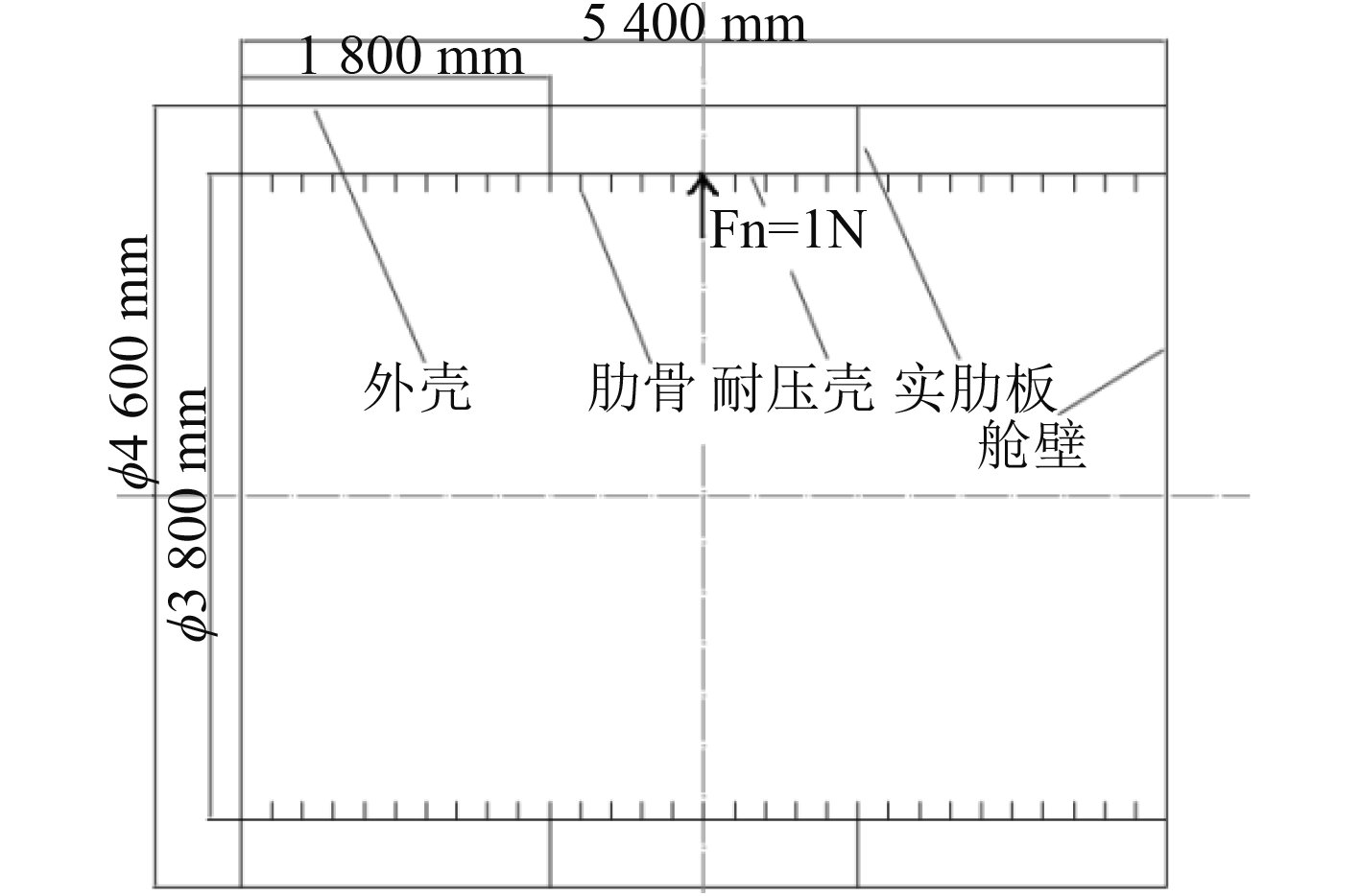图 1 双层圆柱壳模型 Fig. 1 Double cylindrical shell model表 2 圆柱壳结构参数 Tab.2 Data of the cylindrical shell model and material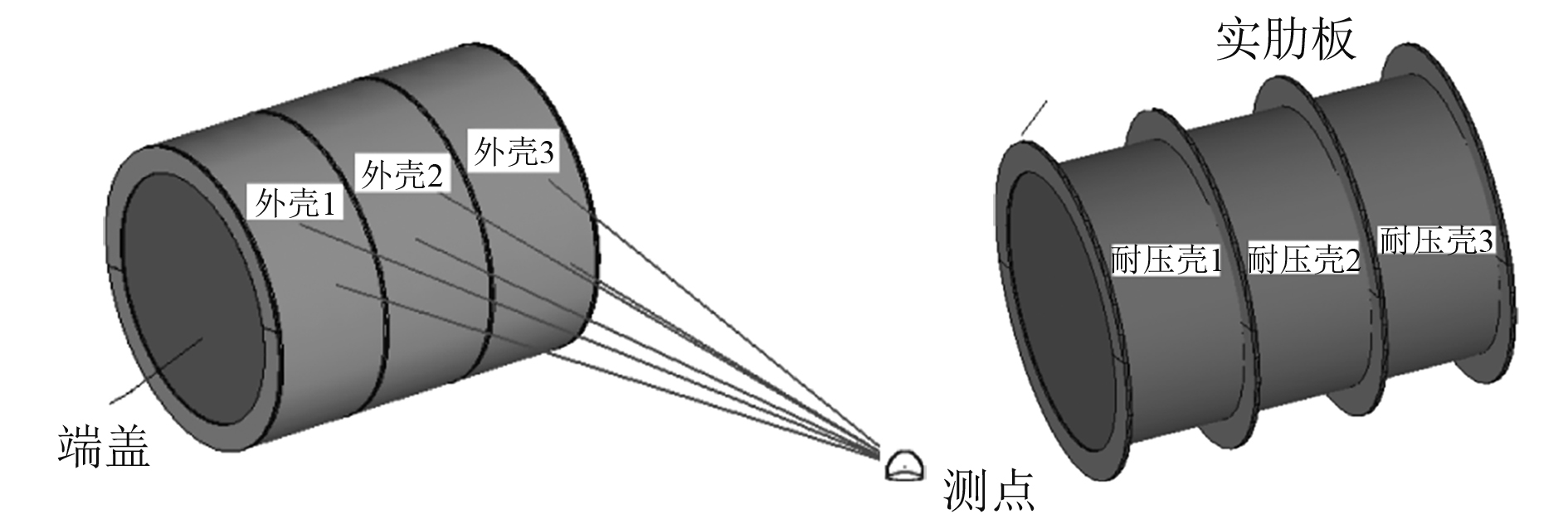图 2 双层圆柱壳的SEA模型 Fig. 2 The SEA model of double cylindrical shell
3 敷设阻尼材料前后双层圆柱壳的振动与辐射噪声对比

3.1 耐压壳敷设阻尼材料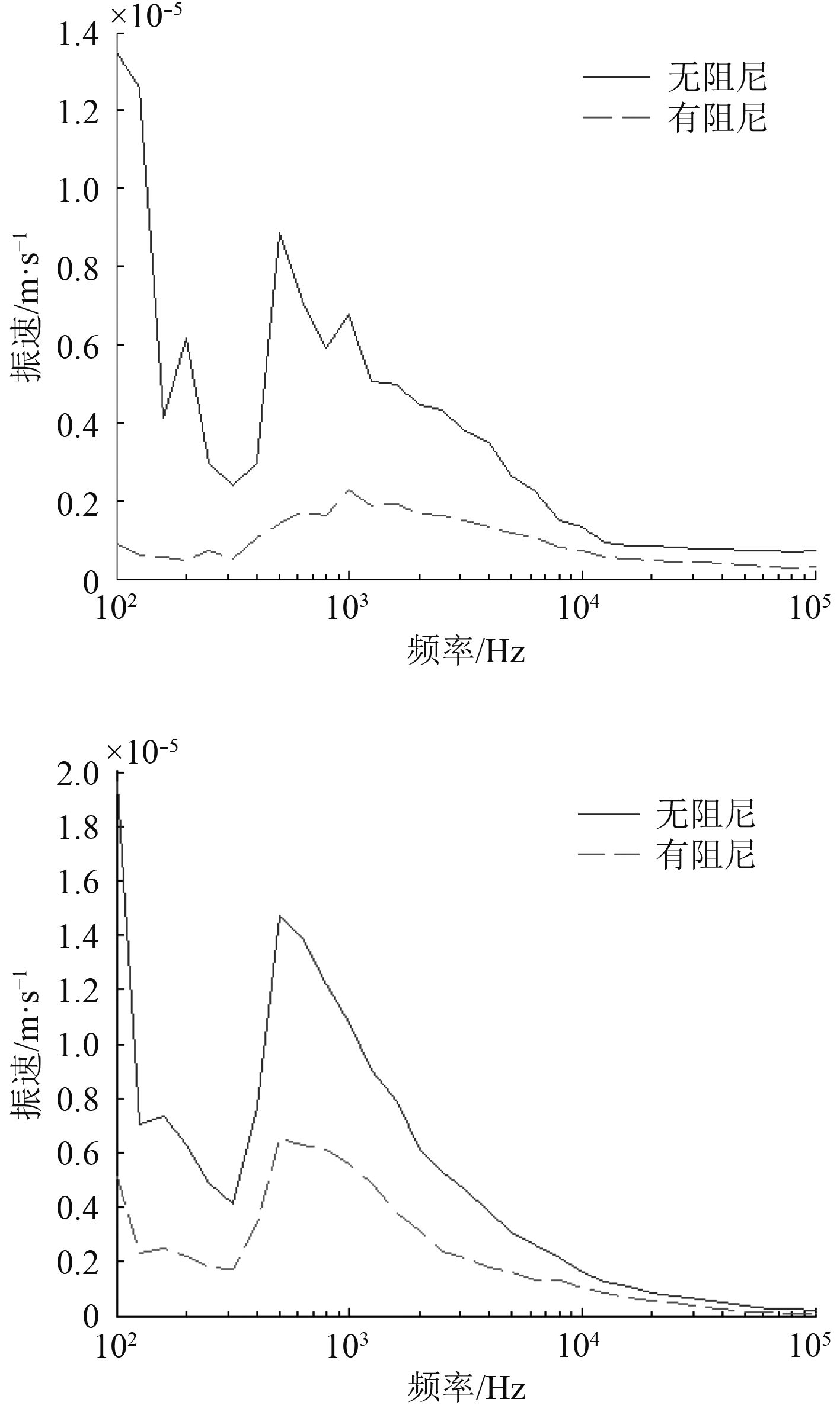图 3 耐压壳2和外壳2的平均振速 Fig. 3 The average speed of the pressure shell and the outer shell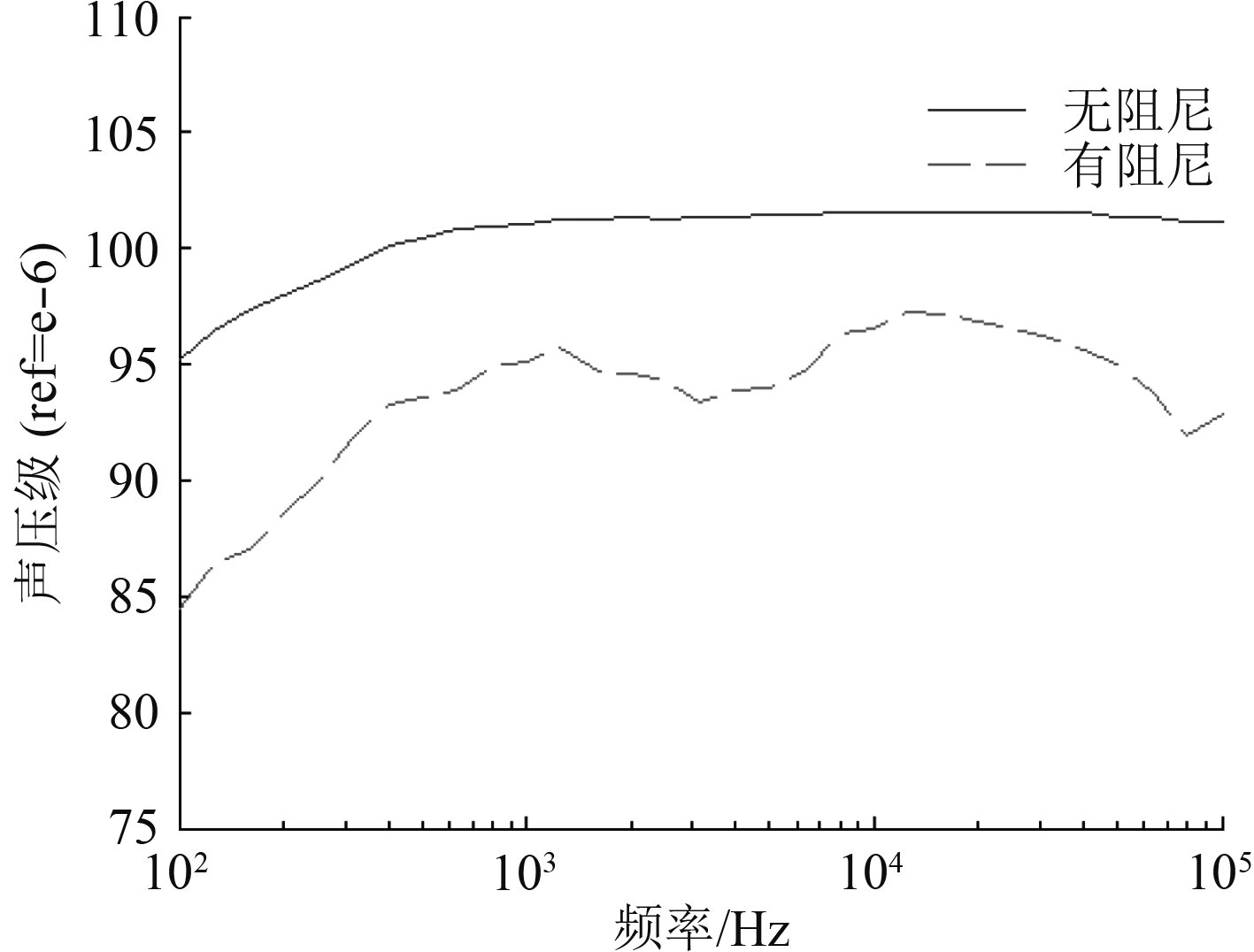图 4 辐射声压级 Fig. 4 Radiation sound pressure level

3.2 外壳敷设阻尼材料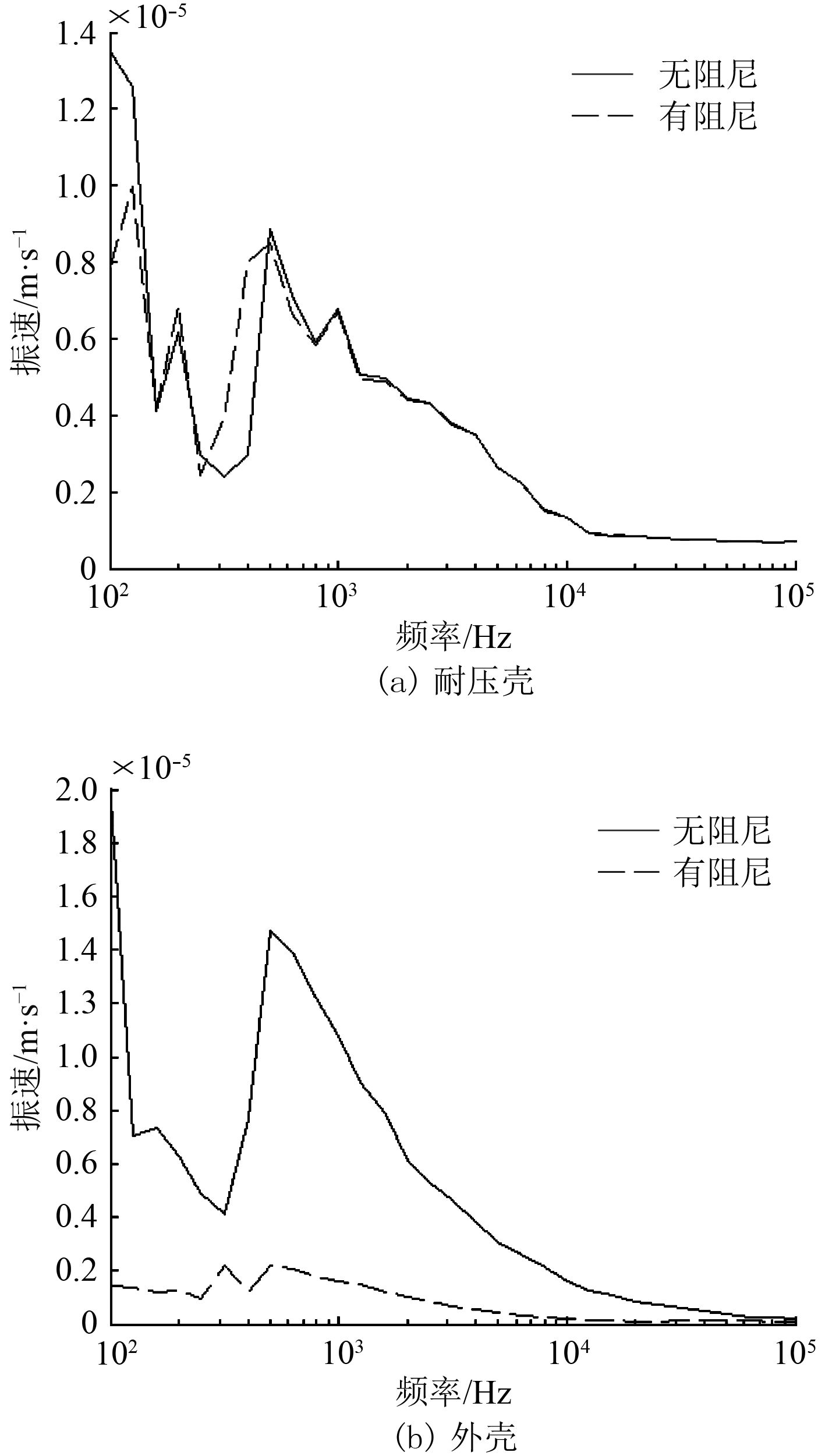图 5 耐压壳2和外壳2的平均振速 Fig. 5 The average speed of the pressure shell and the outer shell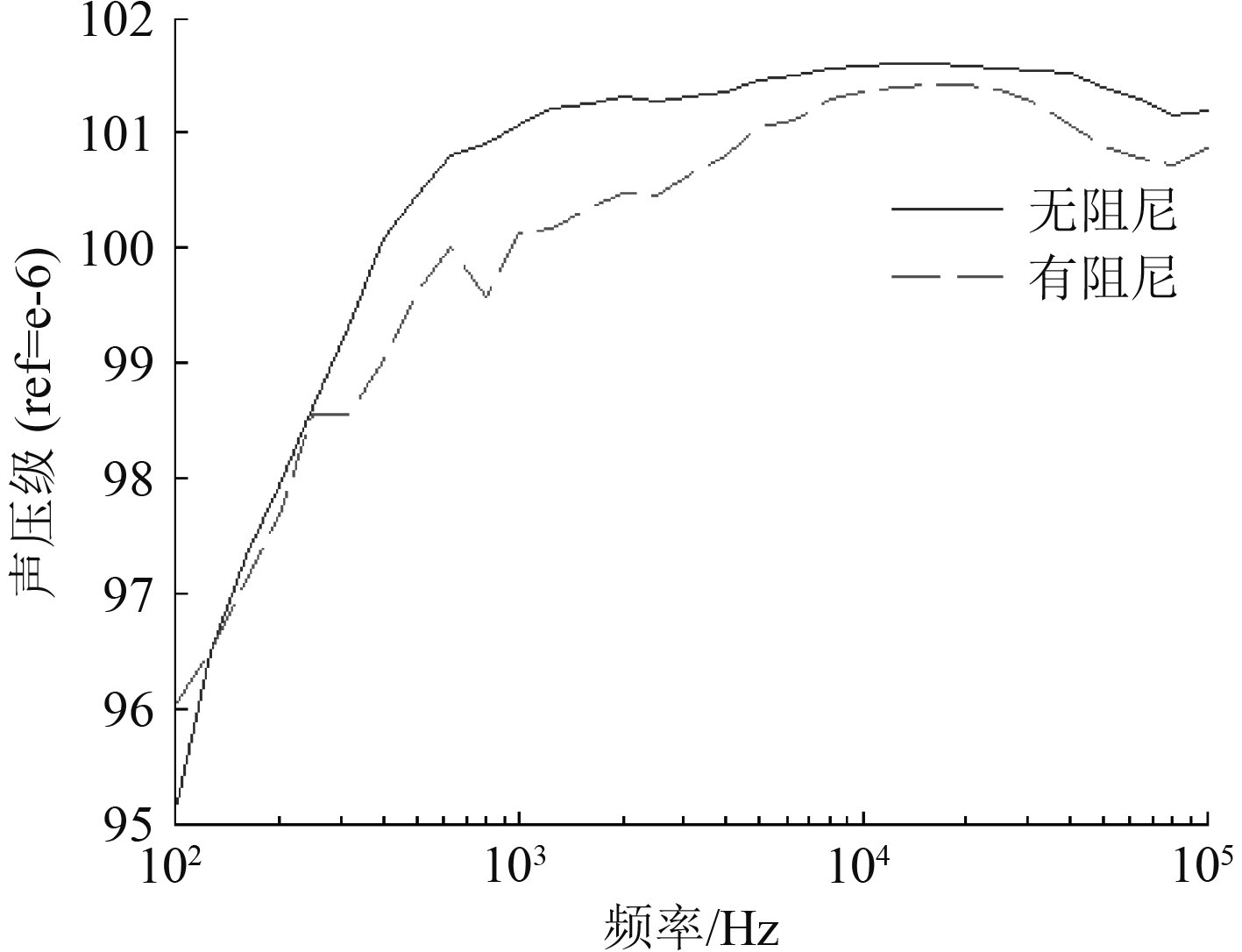图 6 辐射声压级 Fig. 6 Radiation sound pressure level
4 阻尼材料特性对减振降噪效果的影响

4.1 杨氏模量的影响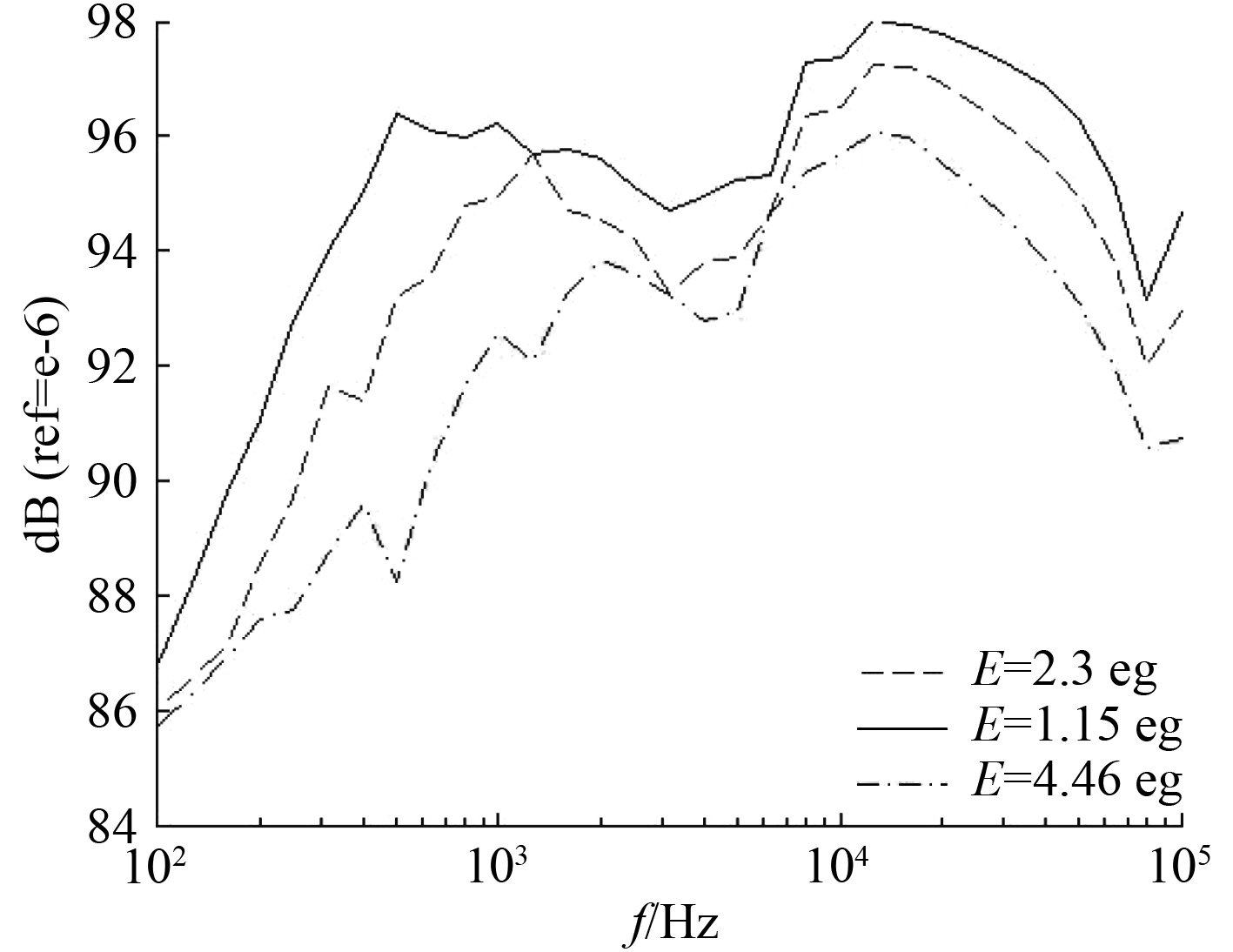图 7 辐射声压级 Fig. 7 Radiation sound pressure level

4.2 损耗因子的影响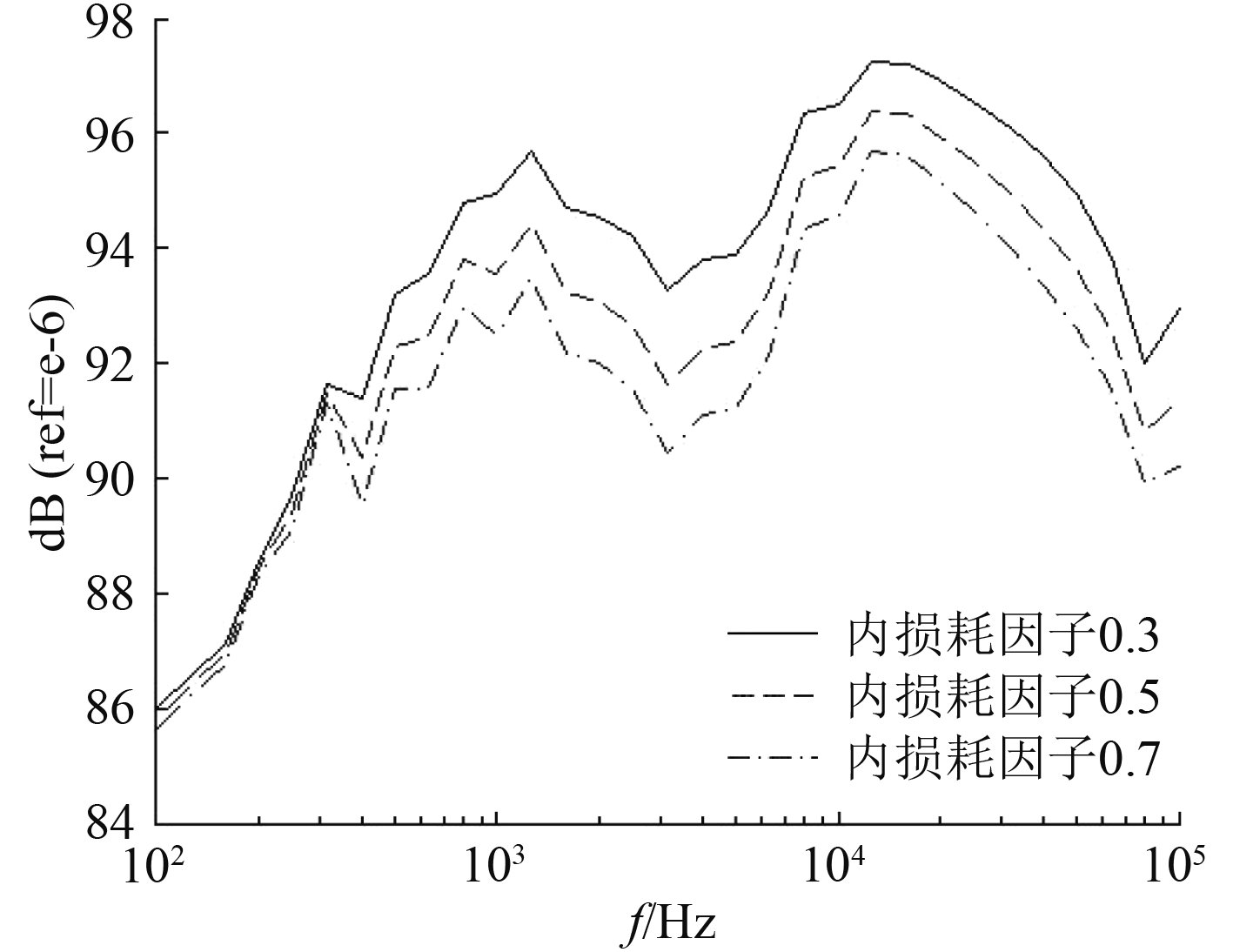图 8 辐射声压级 Fig. 8 Radiation sound pressure level

4.3 厚度的影响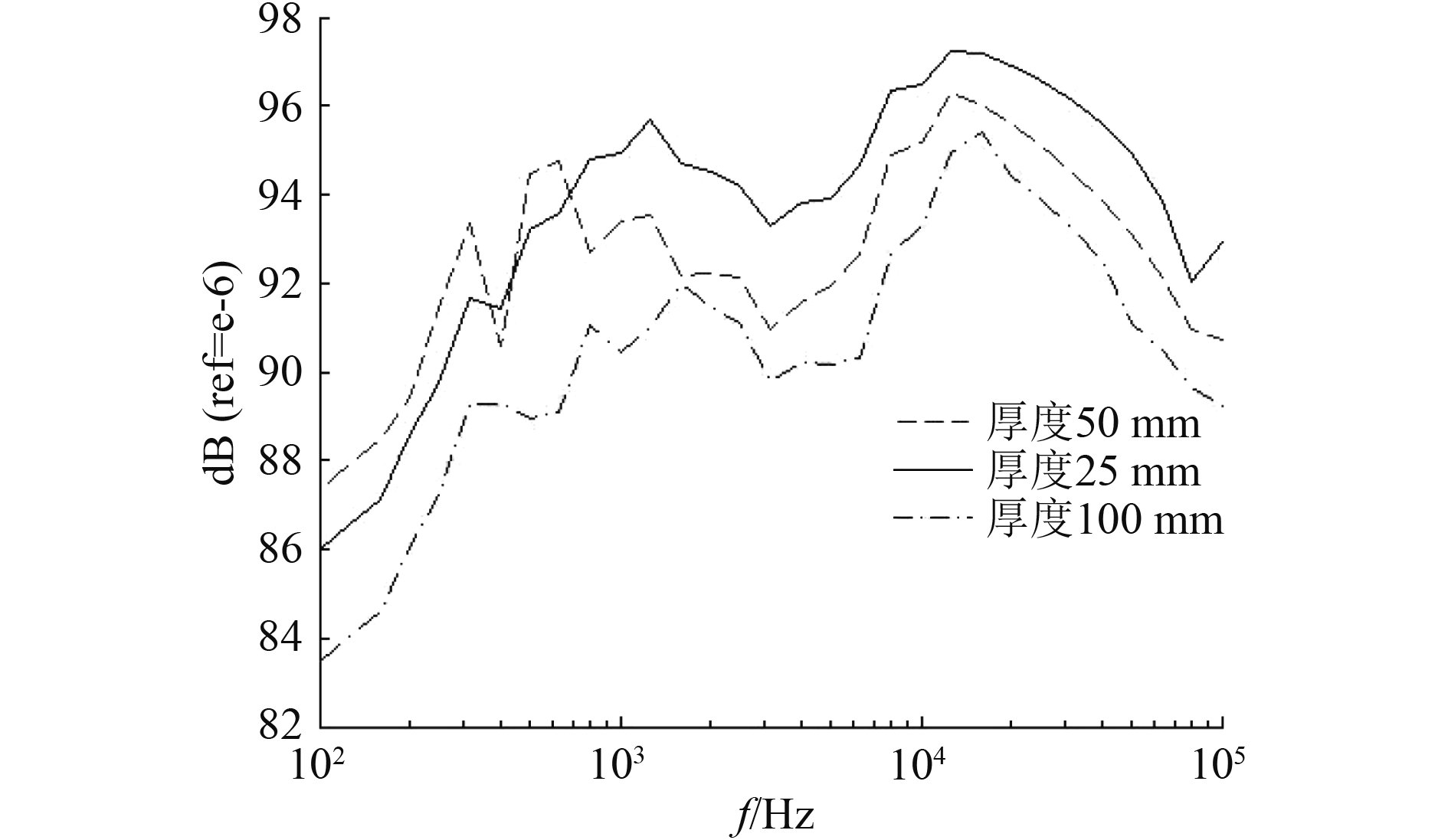图 9 辐射声压级 Fig. 9 Radiation sound pressure level

5 阻尼材料对不同结构形式圆柱壳的降噪效果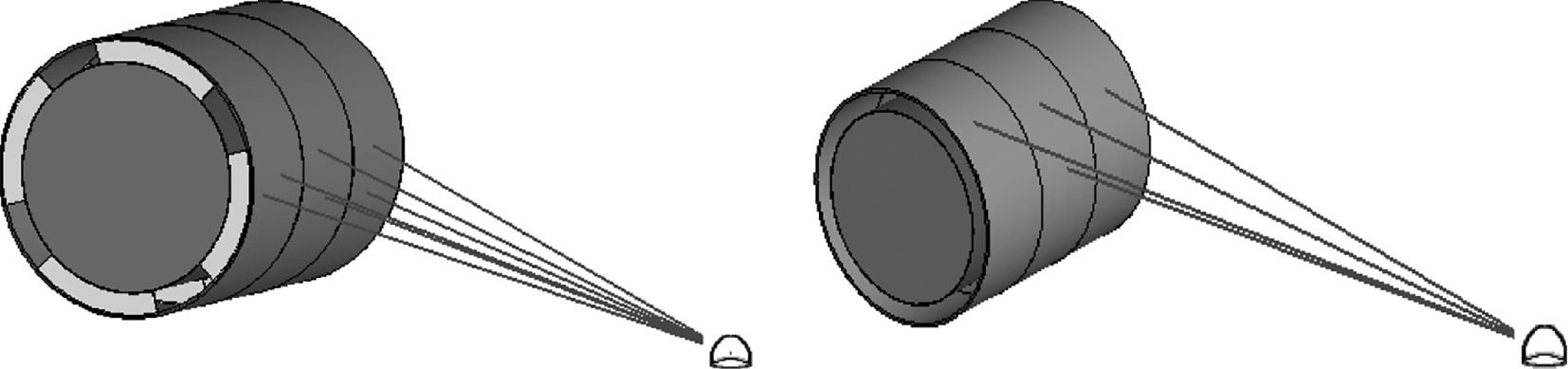图 10 托板连接和无连接件圆柱壳 Fig. 10 Pallet connection and connectionless cylindrical shell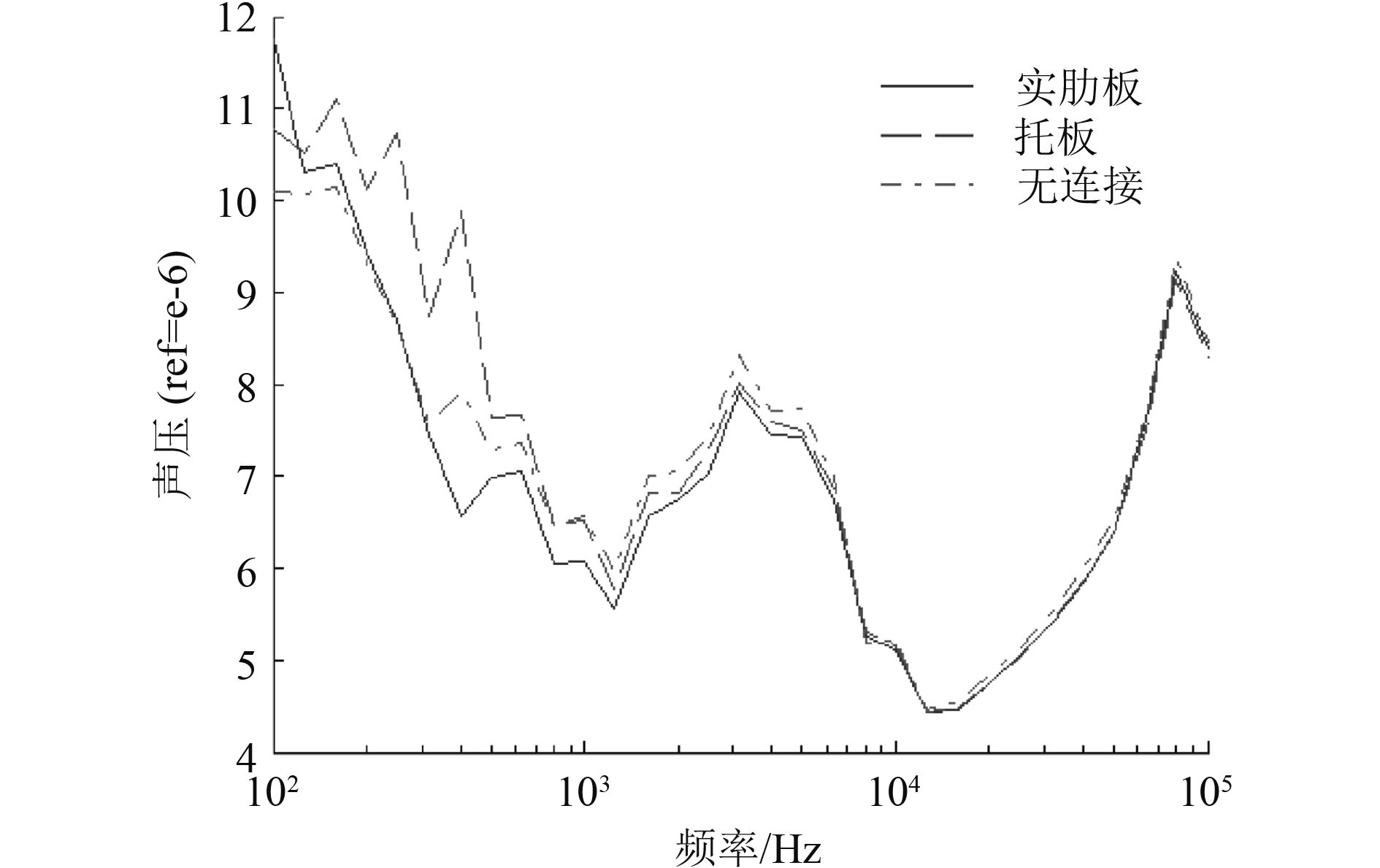图 11 辐射声压级 Fig. 11 Radiation sound pressure level

6 结　语

1）阻尼材料厚度相同时，在耐压壳敷设阻尼材料比在外壳敷设阻尼材料更能抑制结构的振动，降低辐射噪声。

2）增大阻尼材料的杨氏模量能降低结构的辐射噪声；在低频，随着频率的增大，杨氏模量对辐射噪声的影响逐渐增大；在高频，杨氏模量对辐射噪声的影响基本相同。

3）在低频，阻尼材料的损耗因子对结构的辐射噪声影响很小；在高频，增大阻尼材料的损耗因子能够明显降低结构的辐射噪声。

4）在低频，增加阻尼材料的厚度反而可能导致结构辐射噪声的增大；在高频，增加阻尼材料的厚度能够有效的降低结构的辐射噪声。

5）在较低频率时，阻尼材料对托板连接的圆柱壳降噪效果比实肋板和无连接件的圆柱壳好；达到一定频率后对于不同结构形式的圆柱壳，阻尼材料降噪效果相差很小，几乎相同。

  RAMASAMY R, GANESAN N. Vibration and damping analysis of fluid filled orthotropic cylindrical shells with constrained viscoelastic damping[J]. Computers & Structures, 1999, 70(3): 363-376.  陈炜, 骆东平, 张书吉, 等. 敷设阻尼材料的环肋柱壳声辐射性能分析[J]. 声学学报, 2000(1): 27-32. DOI:10.3321/j.issn:0371-0025.2000.01.006  LAULAGNET B, GUYADER J L. Sound radiation from finite cylindrical coated shells, by means of asymptotic expansion of three‐dimensional equations for coating[J]. Journal of the Acoustical Society of America, 1994, 96(96): 277-286.  LAULAGNET B, GUYADER J L. Sound radiation from finite cylindrical shells, partially covered with longitudinal strips of compliant layer[J]. Journal of Sound & Vibration, 1995, 186(186): 723-742.  LAULAGNET B, GUYADER J L. Sound radiation from a finite cylindrical shell covered with a compliant layer[J]. Journal of Vibration & Acoustics, 1991, 113(2): 267.  陈美霞, 骆东平, 彭旭, 等. 敷设阻尼材料的双层圆柱壳声辐射性能分析[J]. 声学学报, 2003(6): 486-493. DOI:10.3321/j.issn:0371-0025.2003.06.002  陈美霞, 骆东平, 周锋, 等. 阻尼材料敷设方式对双层壳体声辐射性能的影响[J]. 声学学报, 2005(4): 296-302. DOI:10.3321/j.issn:0371-0025.2005.04.002  刘向东, 庞福振, 姚熊亮. 敷设阻尼材料的圆柱壳声辐射性能实验分析[J]. 船海工程, 2009(3): 26-31. DOI:10.3963/j.issn.1671-7953.2009.03.009  窦松然. 壳体结构振动及声辐射的粘弹性阻尼控制技术研究[D]. 哈尔滨: 哈尔滨工业大学, 2015.  王献忠, 孙龙泉, 邱忠辉, 等. 部分敷设阻尼材料的水下结构声辐射分析[J]. 振动与冲击, 2012, 31(18): 122-127.  田宝晶. 敷设阻尼层的加肋圆柱壳辐射性能及噪声特性分析[D]. 哈尔滨: 哈尔滨工程大学, 2006.  UNGAR E E. Loss factors of viscoelastically damped beam structures[J]. Journal of the Acoustical Society of America, 1962, 34(8): 1082-1089. DOI:10.1121/1.1918249  姚德源. 统计能量分析原理及其应用[M]. 北京: 北京理工大学出版社, 1995.  LYON R H, DEJONG R G, HECKL M. Theory and application of statistical energy analysis, second edition[J]. Journal of the Acoustical Society of America, 1995, 98(6): 3021.